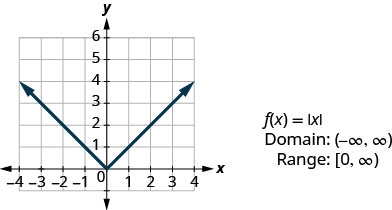# 3.7: 函数图

•• OpenStax
• OpenStax
$$\newcommand{\vecs}{\overset { \rightharpoonup} {\mathbf{#1}} }$$ $$\newcommand{\vecd}{\overset{-\!-\!\rightharpoonup}{\vphantom{a}\smash {#1}}}$$$$\newcommand{\id}{\mathrm{id}}$$ $$\newcommand{\Span}{\mathrm{span}}$$ $$\newcommand{\kernel}{\mathrm{null}\,}$$ $$\newcommand{\range}{\mathrm{range}\,}$$ $$\newcommand{\RealPart}{\mathrm{Re}}$$ $$\newcommand{\ImaginaryPart}{\mathrm{Im}}$$ $$\newcommand{\Argument}{\mathrm{Arg}}$$ $$\newcommand{\norm}{\| #1 \|}$$ $$\newcommand{\inner}{\langle #1, #2 \rangle}$$ $$\newcommand{\Span}{\mathrm{span}}$$ $$\newcommand{\id}{\mathrm{id}}$$ $$\newcommand{\Span}{\mathrm{span}}$$ $$\newcommand{\kernel}{\mathrm{null}\,}$$ $$\newcommand{\range}{\mathrm{range}\,}$$ $$\newcommand{\RealPart}{\mathrm{Re}}$$ $$\newcommand{\ImaginaryPart}{\mathrm{Im}}$$ $$\newcommand{\Argument}{\mathrm{Arg}}$$ $$\newcommand{\norm}{\| #1 \|}$$ $$\newcommand{\inner}{\langle #1, #2 \rangle}$$ $$\newcommand{\Span}{\mathrm{span}}$$$$\newcommand{\AA}{\unicode[.8,0]{x212B}}$$

##### 学习目标

• 使用垂直线测试
• 识别基本函数的图表
• 从函数图中读取信息

1. 评估：ⓐ$$2^3$$$$3^2$$
如果你错过了这个问题，请查看 [链接]
2. 评估：ⓐ$$|7|$$$$|−3|$$
如果你错过了这个问题，请查看 [链接]
3. 评估：ⓐ$$\sqrt{4}$$$$\sqrt{16}$$
如果你错过了这个问题，请查看 [链接]

## 使用垂直线测试图$$\PageIndex{1}$$

##### 示例$$\PageIndex{1}$$ⓐ 由于任何垂直线最多与图形相交一个点，因此该图形是函数的图形。ⓑ 图表上显示的一条垂直线将其交叉成两点。 此图不代表函数。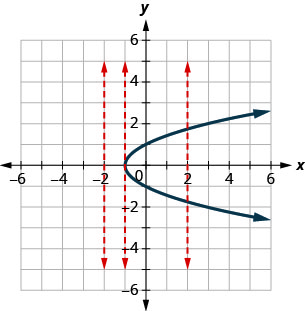##### 示例$$\PageIndex{2}$$ⓐ 是的 ⓑ 不

##### 示例$$\PageIndex{3}$$ⓐ 不 ⓑ 是的

## 识别基本函数的图表

$$y=2x−3$$先前在图中所示的图形与$$f(x)=2x−3$$中所示的图表进行比较。 除了符号之外什么都没有改变。图$$\PageIndex{2}$$
##### 函数图

$\begin{array} {ll} {f} &{\text{name of function}} \\ {x} &{\text{x-coordinate of the ordered pair}} \\ {f(x)} &{\text{y-coordinate of the ordered pair}} \\ \nonumber \end{array}$

##### 线性函数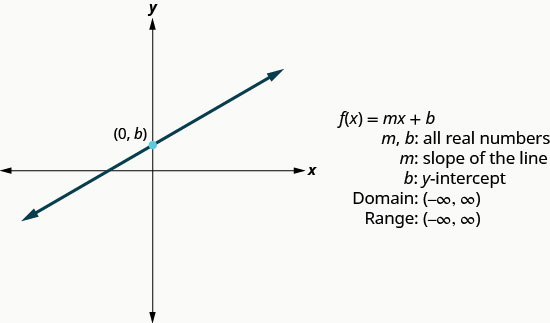##### 示例$$\PageIndex{4}$$

 $$f(x)=−2x−4$$ 我们认为这是一个线性函数。 找出斜率和 y 截距。 $$m=−2$$ $$b=−4$$ 使用斜率截距绘制图形。##### 示例$$\PageIndex{5}$$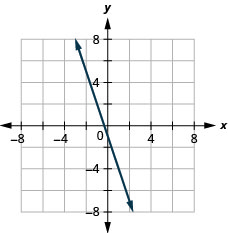##### 示例$$\PageIndex{6}$$##### 常量函数##### 示例$$\PageIndex{7}$$

 $$f(x)=4$$ 我们认为这是一个常量函数。 该图将是一条水平线$$(0,4)$$。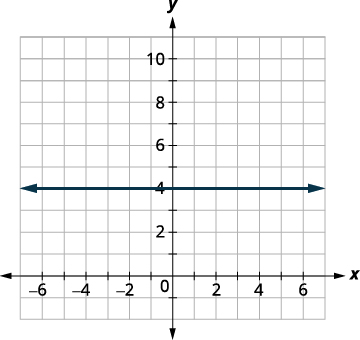##### 示例$$\PageIndex{8}$$##### 示例$$\PageIndex{9}$$##### 身份函数##### 示例$$\PageIndex{11}$$##### 示例$$\PageIndex{12}$$

$$f(x)=−x^2$$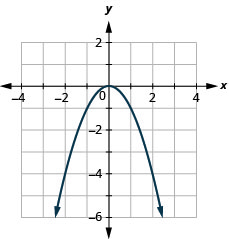##### 方形函数##### 示例$$\PageIndex{14}$$##### 示例$$\PageIndex{15}$$##### 立方体函数##### 示例$$\PageIndex{16}$$

$$f(x)=\sqrt{x}$$##### 示例$$\PageIndex{17}$$##### 示例$$\PageIndex{18}$$##### 平方根函数##### 示例$$\PageIndex{20}$$##### 示例$$\PageIndex{21}$$##### 绝对值函数## 从函数图中读取信息

##### 示例$$\PageIndex{22}$$##### 示例$$\PageIndex{23}$$##### 示例$$\PageIndex{24}$$##### 示例$$\PageIndex{25}$$ⓐ 查找：$$f(0)$$
ⓑ 查找：$$f(32\pi)$$
ⓒ 查找：$$f(−12\pi)$$
ⓓ 在什么时候找出 x 的值$$f(x)=0$$
ⓔ 找到 x 截取。
ⓕ 找到 y 截取。
ⓖ 找到域名。 用间隔符号书写。
ⓗ 找到范围。 用间隔符号书写。

ⓐ 当$$x=0$$，函数在 0 处穿过 y 轴。 所以，$$f(0)=0$$
ⓑ 当$$x=32\pi$$，函数的 y 值为$$−1$$。 所以，$$f(32\pi)=−1$$
ⓒ 当$$x=−12\pi$$，函数的 y 值为$$−1$$。 所以，$$f(−12\pi)=−1$$
ⓓ 该函数在点处为 0$$(−2\pi,0), (−\pi,0), (0,0),(\pi,0),(2\pi,0)$$。 如果$$f(x)=0$$是，则为 x$$−2\pi,−\pi,0,\pi,2\pi$$
x 截获发生在$$y=0$$. 所以 x-截获发生在什么时候$$f(x)=0$$x-interce pts 是$$(−2\pi,0),(−\pi,0),(0,0),(\pi,0),(2\pi,0)$$
y 截获发生在 x=0.x=0 时。 因此 y 截获发生在$$f(0)$$y 截距为$$(0,0)$$
ⓖ 当 x 为从$$−2\pi$$到时，此函数具有一个值$$2\pi$$。 因此，区间表示法中的域为$$[−2\pi,2\pi]$$
ⓗ 此函数值或 y 值从$$−1$$到 1。 因此，以间隔表示法表示的范围为$$[−1,1]$$

##### 示例$$\PageIndex{26}$$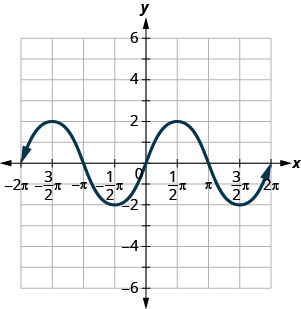ⓐ 查找：f (0) .f (0)。
ⓑ 查找：f (12\ pi) .f (12\ pi)。
ⓒ 查找：f (−32\ pi) .f (−32\ pi)。
ⓓ 在 f (x) =0.f (x) =0 时找出 x 的值。
ⓔ 找到 x 截取。
ⓕ 找到 y 截取。
ⓖ 找到域名。 用间隔符号书写。
ⓗ 找到范围。 用间隔符号书写。

$$f(0)=0$$$$f=(\pi2)=2$$$$f=(−3\pi2)=2$$ ⓓ f$$f(x)=0$$ or$$x=−2\pi,−\pi,0,\pi,2\pi$$$$(−2\pi,0),(−\pi,0),(0,0),(\pi,0),(2\pi,0)$$ ⓕ (0,0) (0,0) ⓖ$$[−2\pi,2\pi]$$$$[−2,2]$$

##### 示例$$\PageIndex{27}$$ⓐ 查找：$$f(0)$$
ⓑ 查找：$$f(\pi)$$
ⓒ 查找：$$f(−\pi)$$
ⓓ 在什么时候找出 x 的值$$f(x)=0$$
ⓔ 找到 x 截取。
ⓕ 找到 y 截取。
ⓖ 找到域名。 用间隔符号书写。
ⓗ 找到范围。 用间隔符号书写。

$$f(0)=1$$$$f(\pi)=−1$$$$f(−\pi)=−1$$ ⓓ f$$f(x)=0$$ or$$x=−3\pi2,−\pi2,\pi2,3\pi2$$$$(−2pi,0),(−pi,0),(0,0),(pi,0),(2pi,0)$$$$(0,1)$$$$[−2pi,2pi]$$$$[−1,1]$$

## 关键概念

• 垂直线测试
• 如果每条垂直线与图形最多相交一个点，则矩形坐标系中的一组点就是函数的图形。
• 如果有任何垂直线在多个点上与图形相交，则该图形不代表函数。
• 函数图
• 函数的图是其所有有序对的图形，即 (x, y) (x, y) 或使用函数表示法 (x, f (x)) (x, f (x))，其中 y=f (x) .y=f (x)。

fxf (x) 有序对坐标的 functionx-corident 的名称 functionxx-corident 的有序对坐标的名称 functionxx-corident

• 线性函数• 常量函数• 身份函数• 方形函数• 立方体函数• 平方根函数• 绝对值函数